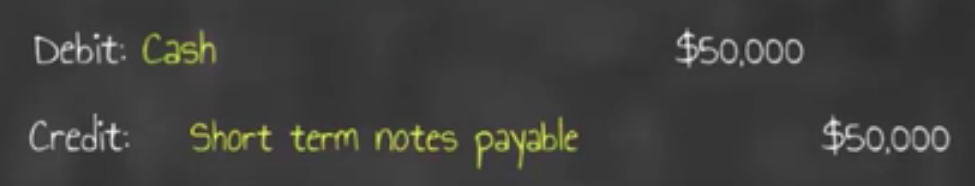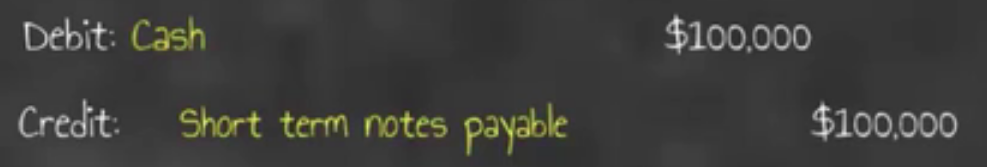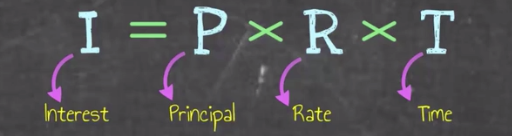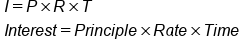+

# Short Term Notes Payable

Author: Sophia Tutorial
##### Description:

Calculate the total interest for a short term notes payable.

(more)### Developing Effective Teams

*No strings attached. This college course is 100% free and is worth 1 semester credit.

37 Sophia partners guarantee credit transfer.

299 Institutions have accepted or given pre-approval for credit transfer.

* The American Council on Education's College Credit Recommendation Service (ACE Credit®) has evaluated and recommended college credit for 32 of Sophia’s online courses. Many different colleges and universities consider ACE CREDIT recommendations in determining the applicability to their course and degree programs.

Tutorial
what's covered
This lesson will cover the topic of short-term notes payable.

Our discussion breaks down as follows:

1. Current Liabilities: A Review
2. Short-Term Notes Payable
3. Interest Payable

## 1. Current Liabilities: A Review

Current liabilities show a company's obligation to pay debts to others within one year or the operating cycle, whichever is shorter; the are they company's short-term debt obligations.

There are several types of current liabilities:

• Accounts payable
• Sales tax payable
• Unearned revenue
• Contingent and uncertain liabilities.

However, in today's lesson, we're going to discuss another current liability called short-term notes payable.

## 2. Short-Term Notes Payable

Short-term notes payable are written agreements to pay a defined sum of money at a specified future date. The key phrase of this definition is "at a specified future date."

Because they are short-term notes payable, they are going to be due within less than a year. So, that specified future date mentioned above is going to be within less than a year.

Short-term notes payable are also interest bearing, so there will be a stated interest rate for our short-term notes payable.

Short-term notes payable are used for things like purchasing equipment, for instance. It's a way to finance that purchase if the company doesn't have the cash on hand immediately.

Let's look at some examples of establishing notes payable.

Example #1
ABC Company borrows \$50,000 from National Bank on January 1, 2012. They sign a 5%, 6-month note.

Look at the key terms underlined above:

• \$50,000 is our specified amount.
• They signed, so there is an agreement.
• 5% is the stated interest rate.
• It's a 6-month note, so it is due in less than a year.

To establish this note payable, the journal entry is going to have a debit to cash in the amount of \$50,000, because the company is receiving cash, and then we're going to credit our short-term notes payable, that current liability account, for \$50,000.Example #2
XYZ Company borrows \$100,000 from Local Bank on January 1, 2012. XYZ Company signs a 6%, 8-month note.

Again, note the underlined terms above:

• \$100,000 = specified sum
• Signs = written agreement
• 6% = stated interest rate
• 8 months = specified future date in less than a year

For the journal entry to establish that note, we would debit our cash for \$100,000, because the company is receiving those funds. Then, we would credit that short-term notes payable current liability account for \$100,000.## 3. Interest Payable

Now let's turn our attention to interest payable. The calculation for determining interest payable reads I equals P times R times T.In this formula, I stands for interest, P stands for principal, or the amount of the loan, R is the stated rate in the written agreement, and T is the length of time that the note is outstanding.

formula
Interest PayableNow, the easiest way to illustrate interest payable is to look at some examples. We'll use the same two examples from above, but this time we're going to calculate interest payable.

Example #1
ABC Company borrows \$50,000 from National Bank and they sign a 5%, 6-month note.

• Principal (P) = \$50,000
• Rate (R) = 5%
• Time (T) = 6 divided by 12, or 6/12

Note, because it's a six-month note and those interest rates are based on an entire year, we would multiply it by the number of months of the note divided by the number of months in the year.Therefore, principle times rate times time gives us interest for this example of \$1,250.

Example #2
XYZ Company borrows \$100,000 from Local Bank on January 1, 2012. XYZ Company signs a 6%, 8-month note.

To calculate interest payable, we're going to go through that same process as above, using our formula.

• Principal (P) = \$100,000
• Rate (R) = 6%
• Time (T) = 8 divided by 12, or 8/12This gives us total interest of \$4,000.

summary
Today we started with a review of current liabilities. Then, we learned about short-term notes payable, which are written agreements to pay a defined sum of money at a specified future date. Lastly, we walked through some calculations of interest payable, using the formula I equals P times R times T.

Source: Adapted from Sophia instructor Evan McLaughlin.

Rating